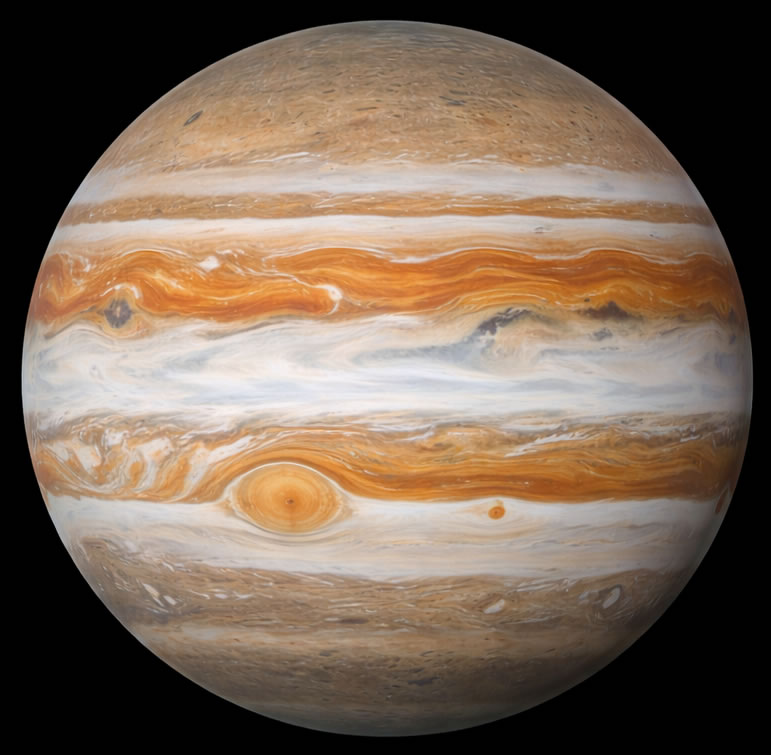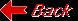Planet JupiterMass (kg) 1.900e+27 Mass (Earth = 1) 3.1794e+02 Equatorial radius (km) 71,492 Equatorial radius (Earth = 1) 1.1209e+01 Mean density (gm/cm^3) 1.33 Mean distance from the Sun (km) 778,330,000 Mean distance from the Sun (Earth = 1) 5.2028 Rotational period (days) 0.41354 Orbital period (days) 4332.71 Mean orbital velocity (km/sec) 13.07 Orbital eccentricity 0.0483 Tilt of axis (degrees) 3.13 Orbital inclination (degrees) 1.308 Equatorial surface gravity (m/sec^2) 22.88 Equatorial escape velocity (km/sec) 59.56 Visual geometric albedo 0.52 Magnitude (Vo) -2.70 Mean cloud temperature -121C Atmospheric pressure (bars) 0.7 Atmospheric composition: Hydrogen 90%, Helium 10%F